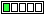All about flooble | fun stuff | Get a free chatterbox | Free JavaScript | Avatarsperplexus dot infoCube Decomposition (Posted on 2018-04-02)A point is picked inside a cube so that it is decomposed into 6 pyramids, the bases being the faces of the cube. The volumes of five of those pyramids are 2, 5, 10, 11, and 14.

What is the volume of the sixth pyramid?

 No Solution Yet Submitted by Danish Ahmed Khan No RatingComments: ( Back to comment list | You must be logged in to post comments.)solution with explanation| Comment 3 of 4 |The volume of a pyramid is the area of the base multiplied by the height divided by 3.

The base of each pyramid in this case is the same: that of one of the faces of the cube. Whatever the edge size of the cube is, the heights of the opposing pyramids (having bases hat are opposite faces of the cube) must add up to the length of an edge of the cube. Thus all pyramid volumes can be arranged in pairs that add up to the same total volume as each of the other pairs.

So two pairs of the given volumes must add to the same sum; the volume of the pyramid that is missing must then complement that of the given volume that is the odd one out, by adding to that volume enough to add up to the sum established by the other two pairs.

2+14 = 16 and 5+11 = 16, so 10 is the odd one out. The missing pyramid must have volume 16 - 10 = 6.

For curiosity's sake, the edge of the cube is found by:

area of each base times sum of two altitudes, divided by 3 is 16:

E^2 * E / 3 = 16
E^3 = 48      (well actually we knew this already by the total volumes)
E = cube root(48) ~=  3.63424118566428

Edited on April 2, 2018, 2:31 pm
 Posted by Charlie on 2018-04-02 14:30:41Please log in:

 Search: Search body:
Forums (4)